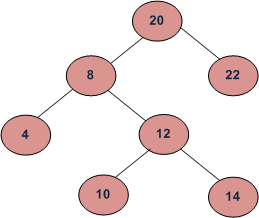TORONTO KIDS COMPUTER CLUB | PMCA Sunday 12:00 Java Homework 20.06.07.
17651

# PMCA Sunday 12:00 Java Homework 20.06.07.

## 12 Jun PMCA Sunday 12:00 Java Homework 20.06.07.

Question:
Given two values n1 and n2, write code to find the lowest common ancestor of number n1 and n2.```Sample Input 1:
10
14```
```Sample Output 1:
12

Explanation:
Both 10 and 14 the children of 12```

```Sample Input 2:
8
14

Sample Output 2:
8

Explanation:
8 is the closest node to both 8 and 14 which is an ancestor of both the nodes```

```Sample Input 3:
10
22

Sample Output 3:
20

Explanation: 20 is the closest node to both 10 and 22
which is an ancestor of both the nodes.```